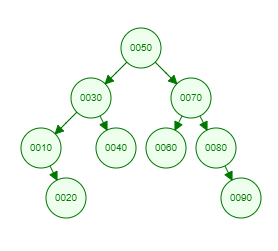Recent Tutorials and Articles
Implementing Binary Search Tree in Java
Published on: 26th October 2018

This tutorial describes how to implement Binary Search Tree in Java containing methods for inserting, deleting and searching a node.

# Introduction to Binary Search Tree

Binary Tree is a data structure where each node can have maximum two child nodes referred as left and right.

Binary Search Tree is a special Binary Tree wherein left child node value is less than parent node value and right child node value is greater than parent node value. This property makes it very efficient data structure as it provides following performance in Big O notation -

1. Insert operation - O(logN)
2. Delete operation - O(logN)
3. Search operation - O(logN)

Here is a sample Binary Search Tree -# Implementing Binary Search Tree in Java

Here is Java code to implement Binary Search Tree along with a main method performing insert and delete operations:

``````package com.aksain.data.structures;

public class BinarySearchTree<T extends Comparable<T>> {

private Node root;

private class Node {
T data;
Node left;
Node right;

private Node(T data) {
this.data = data;
}
}

/**
* Internal method for recursion
*/
private Node doInsert(T data, Node node) {
if(node == null) {
return new Node(data);
}

// If input data is less than node data, modify left else modify right
int result = data.compareTo(node.data);
if(result < 0) {
node.left = doInsert(data, node.left);
} else if(result > 0) {
node.right = doInsert(data, node.right);
}

return node;
}

public void insert(T data) {
root = doInsert(data, root);
}

private T minValue(Node node) {
if(node.left != null) {
return minValue(node.left);
}

return node.data;
}

/**
* Internal method for recursion
*/
private Node doDelete(T data, Node node) {
if(node == null) {
return node;
}
// If input data is less than node data, go left else right
int comp_result = data.compareTo(node.data);
if(comp_result < 0) {
node.left = doDelete(data, node.left);
} else if(comp_result > 0) {
node.right = doDelete(data, node.right);
} else {
if(node.left == null && node.right == null) {
//simply remove the node with no childs
node = null;
} else if(node.left == null) {
// move right child node as node since no left child is present
node = node.right;
} else if(node.right == null) {
// move left child node as node since no right child is present
node = node.left;
} else {
// find minimum value in right subtree and assign that to current node
node.data = minValue(node.right);
node.right = doDelete(node.data, node.right);
}
}
return node;
}

public void delete(T data) {
root = doDelete(data, root);
}

private void doInOrder(Node node) {
if(node == null) {
return;
}
doInOrder(node.left);
System.out.print(node.data + " ");
doInOrder(node.right);
}

public void inOrder() {
doInOrder(root);
System.out.println();
}

public static void main(String[] args) {
final BinarySearchTree<Integer> binarySearchTree = new BinarySearchTree<>();
binarySearchTree.insert(50);
binarySearchTree.insert(30);
binarySearchTree.insert(10);
binarySearchTree.insert(20);
binarySearchTree.insert(40);
binarySearchTree.insert(70);
binarySearchTree.insert(60);
binarySearchTree.insert(80);
binarySearchTree.insert(90);
binarySearchTree.inOrder();

binarySearchTree.delete(80);
binarySearchTree.delete(100);
binarySearchTree.delete(50);

binarySearchTree.inOrder();
}
}
``````Thank you for reading through the tutorial. In case of any feedback/questions/concerns, you can communicate same to us through your comments and we shall get back to you as soon as possible.

Published on: 26th October 2018